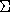advanced## CGAL::partition_is_valid_2

### Definition

Function that determines if a given set of polygons represents a valid partition for a given sequence of points that define a simple, counterclockwise-oriented polygon. A valid partition is one in which the polygons are nonoverlapping and the union of the polygons is the same as the original polygon.

#include <CGAL/partition_is_valid_2.h>

template<class InputIterator, class ForwardIterator, class Traits>
bool
 partition_is_valid_2 ( InputIterator point_first, InputIterator point_beyond, ForwardIterator poly_first, ForwardIterator poly_beyond, Traits traits = Default_traits)
returns true iff the polygons in the range [poly_first, poly_beyond) define a valid partition of the polygon defined by the points in the range [point_first, point_beyond) and false otherwise. Each polygon must also satisfy the property tested by Traits::Is_valid().
 Precondition: Points in the range [point_first, point_beyond) define a simple, counterclockwise-oriented polygon.

### Requirements

1. Traits is a model of the concept PartitionIsValidTraits_2 and the concept defining the requirements for the validity test implemented by Traits::Is_valid().
2. InputIterator::value_type should be Traits::Point_2, which should also be the type of the points stored in an object of type Traits::Polygon_2.
3. ForwardIterator::value_type should be Traits::Polygon_2.

The default traits class Default_traits is Partition_traits_2, with the representation type determined by InputIterator::value_type.

This function requires O(n logn + e loge +i=1p mi) where n is the total number of vertices of the p partition polygons, e is the total number of edges of the partition polygons and mi is the time required by Traits::Is_valid() to test if partition polygon pi is valid.advanced